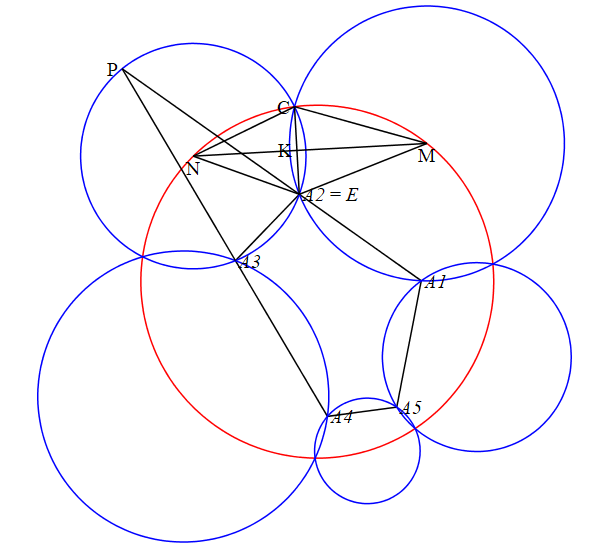:

## Proof of Miquel's theorem with Maple

This post is devoted to the rigorous proof of Miquel's five circles theorem, which I learned about from this question. The proof is essentially very simple and takes only 15 lines of code. The figure below, in which all the labels coincide with the corresponding names in the code, illustrates the basic ideas of the code. First, we symbolically define common points of intersection of blue circles with a red unit circle  (these parameters  s1 .. s5  are the polar coordinates of these points). All other parameters of this configuration can be expressed through them. Then we find the centers  M  and  N  of two circles. Then we find the coordinates of the point  K  from the condition that  CK  is perpendicular to  MN . Then we find the point  and using the result obtained, we easily find the coordinates  of all the points  A1 .. A5. Then we find the coordinates of the point   P  as the point of intersection of the lines  A1A2  and  A3A4 . Finally, we verify that the point  P  lies on a circle with center at the point  N , which completes the proof.Below - the code of the proof. Note that the code does not use any special (in particular geometric) packages, only commands from the Maple kernel. I usually try any geometric problems to solve in this style, it is more reliable,  and often shorter.

restart;
t1:=s1/2+s2/2: t2:=s2/2+s3/2:
M:=[cos(t1),sin(t1)]: N:=[cos(t2),sin(t2)]:
C:=[cos(s2),sin(s2)]: K:=(1-t)*~M+t*~N:
CK:=K-C: MN:=M-N:
t0:=simplify(solve(CK*MN+CK*MN=0, t)):
E:=combine(simplify(C+2*eval(CK,t=t0))):
s0:=s5-2*Pi: s6:=s1+2*Pi:
assign(seq(A||i=eval(E,[s2=s||i,s1=s||(i-1),s3=s||(i+1)]), i=1..5)):
Dist:=(p,q)->sqrt((p-q)^2+(p-q)^2):
LineEq:=(P,Q)->(y-P)*(Q-P)=(x-P)*(Q-P):
Line1:=LineEq(A1,A2):
Line2:=LineEq(A3,A4):
P:=combine(simplify(solve({Line1,Line2},[x,y])))[]:
Circle:=(x-N)^2+(y-N)^2-Dist(N,C)^2:
is(eval(Circle, P)=0);
# The final result

true

It may seem that this proof is easy to repeat manually. But this is not so. Maple brilliantly coped with very cumbersome trigonometric transformations. Look at the coordinates of point  , expressed through the initial parameters  s1 .. s5 :

simplify(eval([x,y], P));  # The coordinates of the point  PProofMiquel.mw﻿